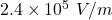## In a given crt, electrons are accelerated horizontally by 7.0kv . they then pass through a uniform electric field e for a distance of 2.4cm

Question

In a given crt, electrons are accelerated horizontally by 7.0kv . they then pass through a uniform electric field e for a distance of 2.4cm , which deflects them upward so they travel 24cm to the top of the screen, 10cm above the center.Estimate the value of E. Neglect the upward distance that electron moves while in the electric field.

in progress 0
2 weeks 2021-08-30T01:26:30+00:00 1 Answers 0 views 0

1. Given that,

Potential energy  = 7.0 kV

Distance = 2.4 cm

Initial horizontal velocity covered 24cm down and new velocity covered 10 cm up.

The final velocity is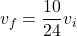The kinetic energy of electron is

Using conservation of energy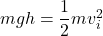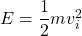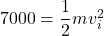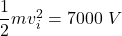When an electron passing through field E experience force F produces change in momentum.

Initial vertical momentum is zero.

We need to calculate the value of E

Using formula of momentum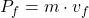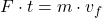Where,F = change in potential energy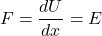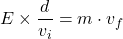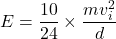Put the value into the formula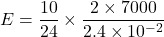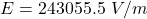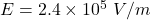Hence, the value of E  is